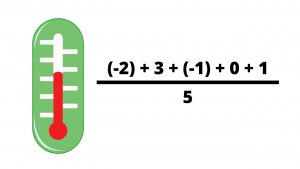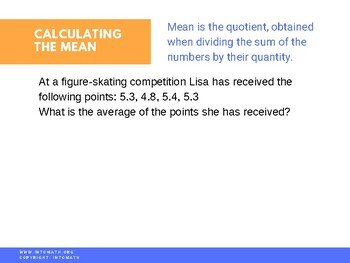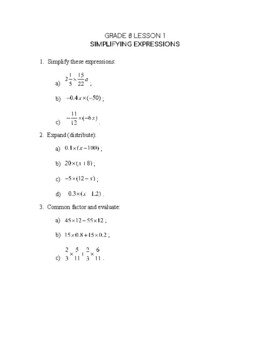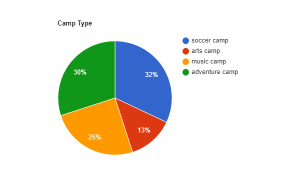Video LessonCA$2.00 In this lesson you will learn what a mean is. You will discover how the mean is related to the average. For example, given a set of numbers: 2, 5, 7, 7, 8 the mean is calculated by first finding the sum of all numbers and dividing the sum by how many numbers there are in a set: (2 + 5 + 7 + 7 + 8)/ 5. The mean is often used in research, academics and in sports.Grade 8 Workbook (18 pages with answers) CA$4.00

The lesson will also teach you how and when to use pie graphs (charts). You often see these graphs used by media to show the relationships between various quantities ( for example, during the elections, bar or pie graphs are used to show the ratings of the candidates.

You will learn how to use bar graphs when representing the relationships between quantities that are not dependent on each other.

How many more students prefer ice cream compared to pasta?What type of camp is the most popular?If 100 % represents 100 children, then how many children attended the adventure camp?What type of camp is the least popular?